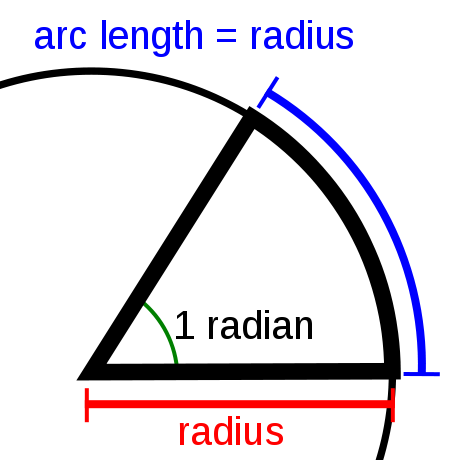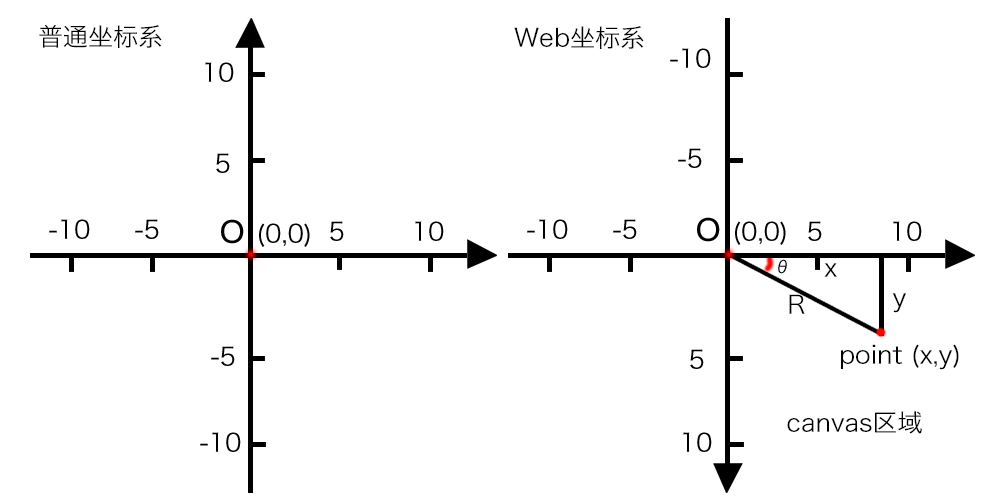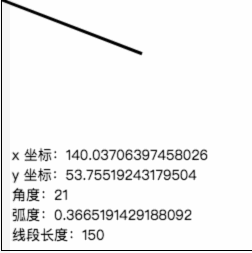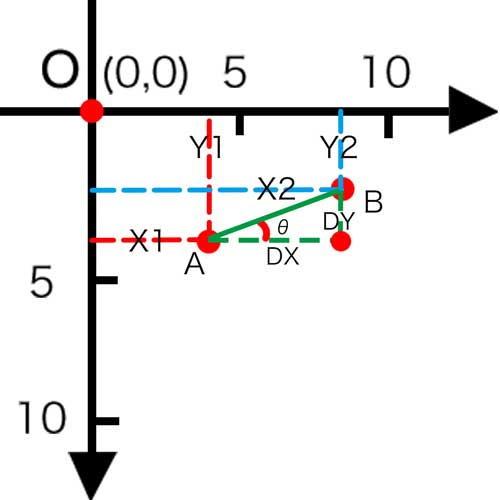# HTML5 Canvas : Application Of Trigonometric Function

## What is trigonometric function?

Trigonometric function is one of the basic elementary functions. It takes the angle (the most commonly used radian system in mathematics, the same below) as the independent variable. It can also be defined equivalently by the length of various line segments related to the unit circle.

In the application of HTML5 canvas, trigonometric function is generally used to calculate the unknown length edge and angle of triangle in canvas.

Common trigonometric functions include sine function, cosine function and tangent function. The javasript API provides math objects that contain these calculations.

``````//Returns the sine of a number.
Math.sin(x)

//Returns the cosine of a number.
Math.cos(x)

//Returns the tangent of a number.
Math.tan(x)

//Where parameter x is a value representing radians
//There are also some commonly used anti trigonometric functions

//Returns the arcsine of a number in radians.
Math.asin(x)

//Returns the arccosine of a number in radians.
Math.acos(x)

//Returns the arctangent of a number in radians.
Math.atan(x)

//Returns the value of the offset angle from the positive x axis to the line between the point (x, y) and the origin, which is a counterclockwise angle in radians.
Math.atan2(y, x)
``````

Through the understanding of Math object in front, we can see that their calculation is related to radian value, and the calculation of radian and angle is inseparable from a constant, that is, PI.

``````//π. That is, the ratio of the circumference of a circle to its diameter. This value is approximately 3.141592653589793.
Math.PI
``````

An arc whose arc length is equal to the radius and whose center angle is 1 radian

According to the definition, the number of radians in a week is 2 π R / r = 2 π, 360 ° angle = 2 π radian, so 1 radian is about 57.3 °, and 1 ° angle = π / 180 radian.Therefore, in JavaScript, the conversion of radians and angles is as follows:

``````Radian value = angle value * math.pi / 180

Angle value = radian value * 180 / math.pi
``````

Through an example, we can intuitively understand the transformation operation process:

``````<html>
<body>
<canvas id="myCanvas" width="250" height="250" style="border: 1px solid">
</canvas>
</body>
</html>
<script>
//Prepare canvas
var canvas = document.getElementById("myCanvas");
var ctx = canvas.getContext("2d");
var x = 40,y = 100;

ctx.lineWidth = 3;
ctx.moveTo(0,0);
ctx.lineTo(x,y);
ctx.stroke();

ctx.font = "14px sans-serif";
//Calculate the radian between the line between the end point and the origin and the X axis
//Convert the angle between the line between the end point and the origin and the X axis
var degrees = Math.atan2(y,x) * 180 / Math.PI;
//According to definition: adjacent edge / hypotenuse = cos (angle)
//Therefore, the length of the segment = hypotenuse = adjacent edge / cos (angle)
var lineLength = x / Math.cos(radians)

ctx.fillText("Y coordinates:" + y, 10, 180);
ctx.fillText("X coordinates:" + x, 110, 180);
ctx.fillText("angle:"+degrees, 10, 200);
ctx.filltext ("line length:" + linelength, 10, 240);
</script>
``````If you write `math. Atan2 (y, x)` as `math. Atan2 (x, y)`, you can also calculate the numerical value, but you need to distinguish that the effective included angle is the included angle formed by the line between the control point and the origin.

## Angles and coordinatesOf course, recalling those trigonometric function formulas that we learned in middle school, we can also backtrack the coordinates of control points through some parameters.

``````//Trigonometric function
sin(θ) = y / R
cos(θ) = x / R
tan(θ) = y / x

//Anti trigonometric function
arcsin(y/R) = θ
arccos(x/R) = θ
arctan(y/x) = θ

//Parameter: x is the adjacent edge, y is the opposite edge, R is the hypotenuse, and θ is the angle
``````

Knowing the mathematical formula, we can calculate the value of abscissa and ordinate, for example:

``````<html>
<body>
<canvas id="myCanvas" width="250" height="250" style="border: 1px solid">
</canvas>
</body>
</html>
<script>
//Prepare canvas
var canvas = document.getElementById("myCanvas");
var ctx = canvas.getContext("2d");

var r = 150
var degrees = 0;

function draw (){
var radians = degrees * Math.PI / 180;

//Calculate the coordinates of the control point through the beveled edge
var x = r * Math.cos(radians);
var y = r * Math.sin(radians);

//Draw the line from the origin to the control point
ctx.lineWidth = 3;
ctx.beginPath();
ctx.moveTo(0,0);
ctx.lineTo(x,y);
ctx.stroke();

ctx.font = "14px sans-serif";
ctx.fillText("x"22352;"26631" + x, 10, 160);
CTX.FillText（“Y”），10，180；
ctx.fillText("35282;" 24230"+degrees, 10, 200);
Ctx.filltext ("line length:" + R, 10, 240);
}

setInterval(function(){
ctx.clearRect(0,0,250,250);
draw ();
if (degrees <=90){
degrees++;
}
},1000)
</script>
``````## Distance between two points

In the above example, we have tried to calculate the length of the line from the control point to the origin, that is, the distance between the two points. In the past study, we know that using Pythagorean theorem can calculate this result more conveniently for us.

If you want to calculate the distance between point A (x1, y1) and point B (x2, y2) of canvas, it is very simple, and the code is as follows:

``````//Distance of X axis between two points
var distanceX = x2 - x1;
//Distance of Y axis between two points
var distanceY = y2 - y1;
//According to Pythagorean theorem, calculate the slanting edge, that is, the distance between two points
var distance = Math.sqrt(distanceX * distanceX + distanceY * distanceY);
``````

## Inclination angle of the line between two pointsIt can be seen from the above figure that tan (θ) = dy / dx, so its angle θ = arctan (dy / dx), and finally it can be concluded that:

``````var angle = Math.atan(distanceY/distanceX);
``````

# You May Also Like